## Interpretation

Figure 11 shows the result for a case where the spacing between slits d is exactly three times the width of the slits. Among many interesting features, two are noteworthy in this result.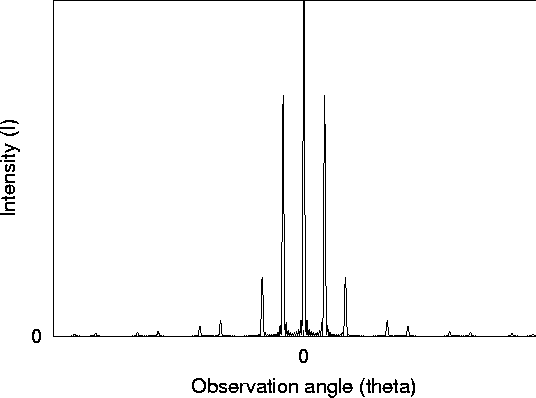Figure 11: Intensity as a function of observation angle for an experiment with N slits of finite width, where N is large (N=10) and the slits are spaced four times further apart than they are wide, d/a=3. Note how this pattern has every third principle maxima (except the central maximum) wiped away.

• Spacing between maxima: Because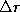is larger (d, the spacing between slits, must be larger than a, the width of the slits, otherwise the slits overlap!), the most rapid oscillations come from the N-slit interference pattern, which then sets the basic pattern. Note that if N is large (N is only ten in the figure), then you see mostly the principle maxima. The little fuzz in the figure hardly visible near zero are the lesser maxima and would become nearly invisible for any large value of N. This is a general feature of the N slit pattern and not particular to the case of finite slits!
• Missing Principle Maxima: The main impact from the finite width of the slits is that it changes the amplitude of the principle maxima. Rather than having equal heights, the height of each maximum is multiplied by the finite-slit factor in Eq. 20. We expect the N-slit pattern (Section 3.3.2) to have principle maxima wherever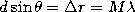, where M is an integer, so that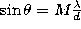. However, these maxima can sometimes be wiped away by the finite-slit diffraction factor in Eq. 20. In particular, the zeros of the finite-slit diffraction pattern occur where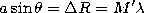, or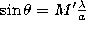, where M' is another integer. Whenever both of these conditions are met at once, we find that the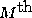expected maximum appears to be missing.

Equating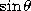in both cases, we find that this happens when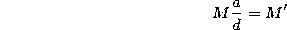is an integer. In our example, a/d=1/3, and so we expect every third principle maximum M (except the one in the center, M=0) to be missing, exactly as in Figure 11!